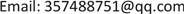1. 引言

1) 将具有大量属性的Web源数据集中的属性依据信息熵和等价类的方法进行属性选择和分类，以减少数据集中的不相关属性的比较；

2) 引入了局部敏感哈希的预处理步骤，将属性匹配归纳法(Attribute-match induction)应用到具有大量属性的Web数据集中，利用属性匹配归纳法进行属性匹配和划分，以减少不相关的属性之间冗余比较；

3) 依据属性匹配和划分的模式信息，利用改进了的基于聚合熵加权图的元分块方法消除了多余比较；

4) 最后对该算法进行了实验验证。

2. 相关工作

3. 相关概念及理论3.1. 相关概念及评价方法

1) Clean-Clean ER：输入两个无重复的实体集合 ε 1 和 ε 2 ，并对来自不同数据源中的实体概要

{ ( p i , p j ) | p i ∈ ε 1 , p j ∈ ε 2 } 进行比较，对Clean-Clean ER最直接的解决方法需要 | ε 1 | × | ε 2 | 次比较；

2) dirty ER：输入含有重复记录的集合 ε ，并对所有可能匹配的实体概要进行了比较。最直接的解决方法是执行 | ε i | 2 次比较。 | ε i | 是一个实体集合 ε i 的基数。

D β 是在块 β 中出现的一组重复实体概要， D δ 是一组在集合 ε 中出现的所有重复实体概要。

3.2. 属性匹配归纳法

{ 〈 a i , a j 〉 | a i ∈ A ε 1 , a j ∈ A ε 2 } ，并利用这些属性对来产生属性分区。即，将属性名称空间 ( A ε 1 × A ε 2 ) 划分成不重叠的子集。

i) 属性值转换：给定两个实体集合 ε 1 , ε 2 ，使用元组 〈 a j , τ ( V a j ) 〉 表示实体集合中的每一个属性， a j ∈ A ε i 是一个属性名， τ 是一个属性值转换函数，生成一组从值 V a j 中派生出来的标记来表示属性 a j 存在于

ε 中。函数 τ 通常是一组文本转换函数(包括标记化、去除禁用词、词元化等)。给定一个转换函数 τ ，

ii) 属性表示模型：用矢量 T i 来表示每一个属性 a i ，每一个值 v i n ∈ T i 与元素 t i ∈ T A 相关联。如果 t n ∉ τ ( V a i ) ，那么 v i n 就等于0。如果 t n ∈ τ ( V a i ) ，那么 v i n 就使用加权函数计算的值表示，比如：加权函数可采用TF-IDF(tn)，或者在 τ ( V a i ) 中的元素 t n 使用二进制表示(即：如果 t n ∈ τ ( V a i ) 那么 V i n = 1 ，否则 V i n 为

0)。例如：属性值转换 是将值转换成标记，采用二进制表示这些标记，然后把属性集被表示为一个矩阵：列对应于属性；行对应于实体集合中出现的可能的标记；如果标记 t n 出现在属性 a i 中，每一个元素表示为 V i n = 1 否则表示为0。

iii) 相似度测量：对于每一个可能的属性对 ( a j , a k ) ∈ ( A ε 1 × A ε 2 ) 的相似度，是通过测量矢量 T i 和 T k 的相似性来比较的(比如：相似性函数可采用Dice，Jaccard，Cosine)。注意，相似性度量方法必须与属性模型表示兼容；例如，Jaccard的相似度不能与TF-IDF的权值同时使用。

iv) 聚类算法：该算法输入属性表示模型及其实体概要的相似度，对它们的属性名进行非重叠分区，它的输出是属性分区。

3.3. 元分块

4. 松散模式感知元分块方法模型

4.1. 单数据源属性选择

4.1.1. 分类属性选择

H ( X ) = − ∑ i = 1 n     p ( x ) log p (x)

4.1.2. 值属性选择

Part of the household tabl

4.2. 多数据源松散属性匹配归纳法

4.2.1. 局部敏感哈希预处理步骤

4.2.2. 松散属性匹配归纳法

1) edges←{}sim←Map

2) Max←Map Cand←Map

//属性匹配最大相似度

3) foreach a i ∈ A 1 , a j ∈ A 2 do

4) sim←( i,a j>,similarity( τ i , τ j ))

5) if Sim.get( i,a j>) > Max.get(a i) then

6) Max←(ai,sim)

7) if Sim.get( i,a j>) > Max.get(a j) then

8) Max←(aj,sim)

//属性匹配，找出候选集

9) foreach a i ∈ A 1 , a j ∈ A 2 do

10) if Sim.get( i,a j>) ≥ (a∙Max.get(a i)) then

11) Candidates←(ai,aj)

12) if Sim.get( i,a j>) ≥ (a∙Max.get(a j)) then

13) Candidates←(aj,ai)

14) foreach a i ∈ A 1 ， a j ∈ Candidatesget(ai) do

15) if a i ∈ Candidatesget(aj) then

16) edges← i,a j>

17) K←getConnectedComponentsGrThan1(edges)

return K

4.3. 松散模式感知元分块方法

4.3.1. 分块图加权

H ¯ ( C k ) = 1 | C k | ⋅ ∑ A i ∈ C k H (Aj)

h ( β j ) = 1 | β j | ⋅ ∑ b i ∈ β j h (bi)

h ( b i ) = H ¯ ( C k ) 是与一个分块键 b i → C k 相关联的熵。

Associated tables of p u , p
p v ( p 3 )¬ p v ( p 3 )
p u ( p 1 )n 11 ( 4 )n 12 ( 2 )n 1 + ( 6 )
¬ p u ( p 1 )n 21 ( 3 )n 22 ( 3 )n 2 + ( 6 )
n + 1 ( 7 )n + 2 ( 5 )n + + ( 12 )

W u v = χ 2 ⋅ h ( β u v ) = ∑ i ∈ { 1 , 2 } ∑ j ∈ { 1 , 2 } n i j − u i j u i j ⋅ h ( β u v )

4.3.2. 图修剪

θ i ¯ = M c ，在这里M是最大的权值，c是任意常数。在真实数据集中显示为具有有效性的c值是c = 2；

c值越高实现更高的召回率(PC)，但代价是牺牲精准度(PQ)。

5. 实验评估5.1. 数据集

Statistics of information extracted from real estat

...............

5.2. 实验方法

Blocking method
NameDescription

5.3. 实验结果及分析

Experimental result

P (%)R (%)F1 (%)P (%)R (%)F1 (%)P (%)R (%)F1 (%)

6. 结束语